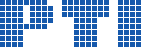Polish Information Processing Society# Implementation of a distributed parallel in time scheme using PETSc for a Parabolic Optimal Control Problem

## Juan Cáceres, Benjamín Barán, Christian Schaerer

Citation: Proceedings of the 2014 Federated Conference on Computer Science and Information Systems, M. Ganzha, L. Maciaszek, M. Paprzycki (eds). ACSIS, Vol. 2, pages 577586 ()

Full text

Abstract. This work presents a parallel implementation of the Parareal method using Portable Extensible Toolkit for Scientific Computation (PETSc). An optimal control problem of a parabolic partial differential equation with known boundary conditions and initial state is solved, where the minimized cost function relates the controller \$v\$ usage and the approximation of the solution \$y\$ to an optimal known function \$y^*\$, measured by \$\|y\|\$ and \$\|y\|\$, respectively. The equations that model the process are discretized in space using Finite Elements and in time using Finite Differences. After the discretizations, the problem is transformed to a large linear system of algebraic equations, that is solved by the Conjugate Gradient method. A Parareal preconditioner is implemented to speed up the convergence of the Conjugate Gradient.